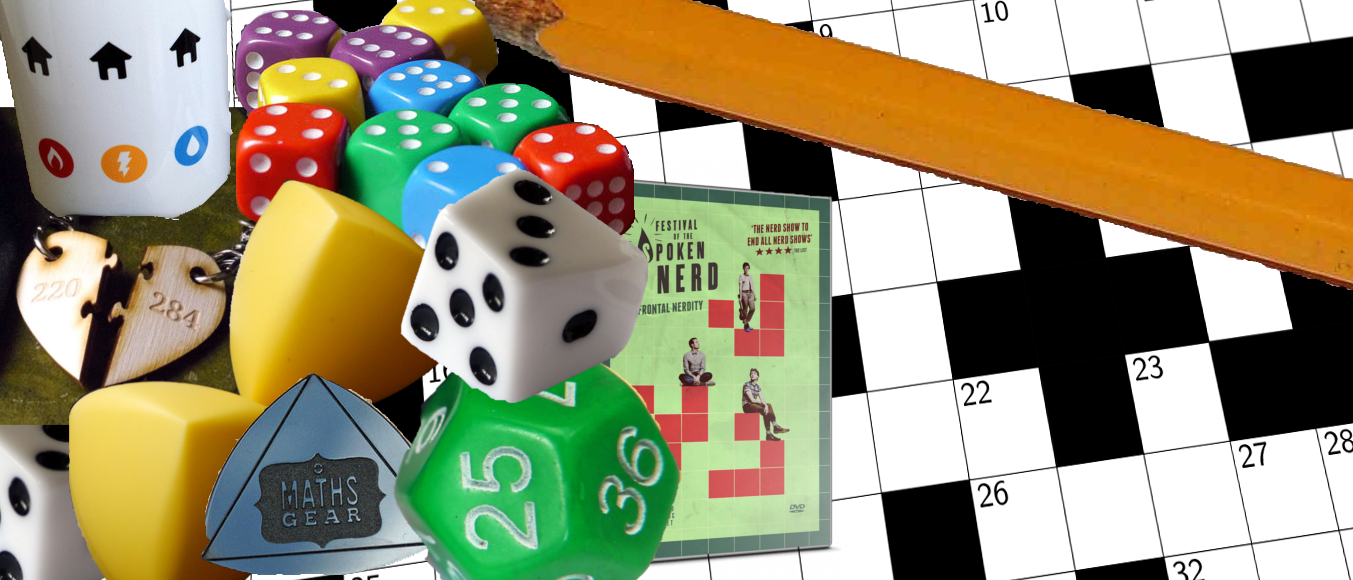# Prize crossnumber, Issue 05

Win £100 of Maths Gear goodies by solving our famously fiendish crossnumberOur original prize crossnumber is featured on pages 58 and 59 of Issue 05.

### Rules

• Although many of the clues have multiple answers, there is only one solution to the completed crossnumber. As usual, no numbers begin with 0. Use of Python, OEIS, Wikipedia, etc. is advised for some of the clues.
• One randomly selected correct answer will win a £100 Maths Gear goody bag. Three randomly selected runners up will win a Chalkdust t-shirt. The prizes have been provided by Maths Gear, a website that sells nerdy things worldwide, with free UK shipping. Find out more at mathsgear.co.uk
• To enter, submit the sum of the across clues via this form by 22 July 2017. Only one entry per person will be accepted. Winners will be notified by email and announced on our blog by 30 July 2017.

### Crossnumber

Crossnumber #5, set by Humbug:

### Clues

#### Across

• 1. The last five digits of the square of this number are equal to this number. (5)
• 5. The square of this number is one more than the product of four consecutive integers. (3)
• 7. This number is equal to the sum of the factorials of its digits. (3)
• 9. A number $a$ such that the equation $3x^2+ax+75$ has a repeated root. (2)
• 10. The geometric mean of 27D and 6D. (3)
• 12. The 2nd, 4th, 6th, 8th, and 10th digits of this number are the highest common factors of the digits either side of them. (11)
• 13. The geometric mean of 6D and 4D. (5)
• 14. This number contains each of the digits 1 to 9 exactly once. The number formed by the first $n$ digits of this number is divisible by $n$ (for all $n$). (9)
• 15. The geometric mean of 4D and 5D. (7)
• 17. Each digit of this number (after the first two) is a digit in the product of the previous two digits of this number. This number’s second digit is 9. (7)
• 18. An integer. (9)
• 20. A number, $n$, such that $n$, $n+1$, $n+2$, $n+3$ and $n+4$ all have the same number of factors. (5)
• 21. A multiple of 5,318,008. (11)
• 24. A multiple of 9 whose digits are all even. (3)
• 26. The number of the across clue whose answer is 26. (2)
• 28. In a base other than 10, this number can be written as 1,000,000. (3)
• 29. A number $n$ such that $n$, $n+1$ and $n+2$ are all the sum of two distinct square numbers. (3)
• 30. The sum of this number’s factors is 100,000. (5)

#### Down

• 1. This number is equal to the last four digits of its square. (4)
• 2. A number whose square and cube between them use each digit 0 to 9 exactly once. (2)
• 3. This number can be written as the sum of the fourth powers of two rational numbers, but it cannot be written as the sum of the fourth powers of two integers. (4)
• 4. The geometric mean of 13A and 15A. (6)
• 5. The geometric mean of 15A and 18A. (8)
• 6. The geometric mean of 10A and 13A. (4)
• 7. This number is a multiple of 3D but not a multiple of 4. Its digits add up to 27D. (12)
• 8. This number can be made by concatenating two other answers in this crossnumber. (10)
• 11. The number of squares (of any size) on a 13,178-by-13,178 chessboard. (12)
• 13. A multiple of both 6D and 20A. (10)
• 16. The sum of this number’s first five digits is one more than the sum of its last three digits. (8)
• 19. The square of this number is a palindrome. (6)
• 22. The number of 41-dimensional sides on a 43-dimensional hypercube. (4)
• 23. Each digit of this number is either a factor or a multiple of the previous digit. (4)
• 25. A number of the form $n^2+2^n$. (4)
• 27. The total number of zeros, threes, sixes, and nines that appear in the completed crossnumber. (2)